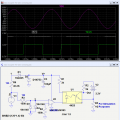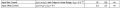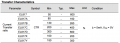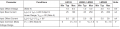# Zerocross test issue

#### crutschow

Joined Mar 14, 2008
25,680
If I count D2, the current is about 50nA. It is very small. How do I apply for it?
What do you mean I didn't include the 1.3V forward drop of the input diode which subtracts from the voltage drop across the resistor 3k?
Is it 5.23V - 1.3V = 3.93V? I already know the current is about 60mA. Please let me know.
The other issue is that 0.33uF is so big size and not cost efficiency. Can we use high value resistors to made voltage divider?
50nA is the D2 leakage current?
The forward current, whcn it clamps the voltage is about 18mA peak.

Yes, the voltage across the resistor in series with the opto input is indeed 6.5V (from my simulation) - 1.3V = 5.2V.
This gives a current of 5.2V /3k = 1.73mA.
How do you "know" that the current is 60mA?Yes, you could use an 8K resistor in place of C2 but it would need to dissipate 1.7W so should be a 3W device.
Not sure how that compares in size and cost with a 0.33uF, 500V cap.

#### Benengineer

Joined Feb 6, 2016
133
50nA is the D2 leakage current?
The forward current, whcn it clamps the voltage is about 18mA peak.

Yes, the voltage across the resistor in series with the opto input is indeed 6.5V (from my simulation) - 1.3V = 5.2V.
This gives a current of 5.2V /3k = 1.73mA.
How do you "know" that the current is 60mA?Yes, you could use an 8K resistor in place of C2 but it would need to dissipate 1.7W so should be a 3W device.
Not sure how that compares in size and cost with a 0.33uF, 500V cap.
50uA is the D2 reverse current. The forward current is about 150mA for the D2 in data sheet. How did you get 18mA peak?
So, the current in the path from C2 to R1 is 1.73mA. The power loss is 5.2Vx0.00173A = 0.09W. The current in the path from IN to the inverting terminal of U2 is 120Vx√2-0.7V/100k = 0.002A. The power loss is about 120Vx√2 x 0.002A = 0.339W. So, the total power is about 0.09W + 0.339W = 0.429W. Are these results right?

Thanks

#### crutschow

Joined Mar 14, 2008
25,680
50uA is the D2 reverse current. The forward current is about 150mA for the D2 in data sheet. How did you get 18mA peak?
So, the current in the path from C2 to R1 is 1.73mA. The power loss is 5.2Vx0.00173A = 0.09W. The current in the path from IN to the inverting terminal of U2 is 120Vx√2-0.7V/100k = 0.002A. The power loss is about 120Vx√2 x 0.002A = 0.339W. So, the total power is about 0.09W + 0.339W = 0.429W. Are these results right?
The maximum forward diode current is 150mA which is unrelated to that actual diode current.
I measured 18mA peak from the simulation.

You power calculations seem correct.

Here's a modification of the circuit to avoid the capacitors and use a resistor, rectifier, and zener to give a 6V supply for the comparator and opto input.
R6 needs to be a 1W resistor (2W preferably) to operate with a 277V input.#### Attachments

• 2.7 KB Views: 0
Last edited:

#### Lestraveled

Joined May 19, 2014
1,946
@crutschow
I thought your idea of the capacitive voltage divider very good, in that, very little heat was dissipated. Why couldn't you incorporate a zener into that design instead of dissipating 1/2 watt with R6. (Please excuse my armchair quarterbacking.)

#### crutschow

Joined Mar 14, 2008
25,680
@crutschow
I thought your idea of the capacitive voltage divider very good, in that, very little heat was dissipated. Why couldn't you incorporate a zener into that design instead of dissipating 1/2 watt with R6. (Please excuse my armchair quarterbacking.)
It's not related to voltage regulation.
He said the .33uF, 500V capacitor is too large and expensive (post #40) so I came up with an alternate that didn't use that capacitor.
I personally prefer the capacitor divider approach also.

#### Benengineer

Joined Feb 6, 2016
133
Okay, one more try.
I was able to get the circuit to operate reasonably well with a C2 value of 0.1μF which should be significantly smaller and cheaper, such as this one.

View attachment 105968
Thank you. Why do you use R4 as 100k? Can we directly connect non-inverting terminal to ground?
You changed R2 into 5K, why?
Thanks

#### crutschow

Joined Mar 14, 2008
25,680
The value of R4 of 100k is somewhat arbitrary.
It's a tradeoff between power dissipation and error due to the comparator input bias current.

I added 100k at the non-inverting input to reduce bias error.
If the the input resistors are equal in value then the voltage error due to input bias current is equal to only the bias current offset, not the total bias current.

I changed R2 to 5k to make sure the opto input current is sufficient to drive the output for an opto with minimum gain, and 5k still gave a sufficiently fast response time.

#### Benengineer

Joined Feb 6, 2016
133
The value of R4 of 100k is somewhat arbitrary.
It's a tradeoff between power dissipation and error due to the comparator input bias current.

I added 100k at the non-inverting input to reduce bias error.
If the the input resistors are equal in value then the voltage error due to input bias current is equal to only the bias current offset, not the total bias current.

I changed R2 to 5k to make sure the opto input current is sufficient to drive the output for an opto with minimum gain, and 5k still gave a sufficiently fast response time.
I don't understand the statement "I added 100k at the non-inverting input to reduce bias error". How does it reduce bias error? Can you explain "If the the input resistors are equal in value then the voltage error due to input bias current is equal to only the bias current offset, not the total bias current."
When you say R2 choose 5k, it is because minimum gain can be gotten. what is gain value in the case? Is it R2/R1? Since we calculated forward current in diode is 6.5V (from my simulation) - 1.3V = 5.2V; 5.2V /3k = 1.73mA. So, the Ic = 1.73mA * R2/R1( 5K/3K)= 2.883mA. Why do we need minimum gain to make sure sufficient input current to drive opto output? I am hungary for those knowledge since i am a beginner. I am very interested in the project. I think this is the best forum I have ever had. I am very appreciate your help.

Ben

#### crutschow

Joined Mar 14, 2008
25,680
If you look at the data sheet for the LM393 it gives values for input bias current which goes in/out of both inputs and the input offset, which is the difference in current between the two inputs:This bias current through any input resistance will cause a voltage error at the input.
However, if you put the same resistance at both inputs then this voltage error is cancelled if both bias currents were identical, since the same voltage would appear at both inputs.

There can be a slight difference between these input currents, however, called the Input Offset Current and the matched resistors can't cancel that.
The input offset voltage is then equal to this Offset current times the value of the two matched input resistors, but that's much less (typically a factor of 8 for the LM393) then would be caused by the input bias going through one resistor.

The opto gain I'm talking about is current-gain (current transfer ratio) between the input and output as shown in the data sheet, not the voltage-gain:You want to keep the current gain in your circuit below the minimum value to insure that the circuit will work for worst-case devices.

Last edited:

#### Benengineer

Joined Feb 6, 2016
133
If you look at the data sheet for the LM393 it gives values for input bias current which goes in/out of both inputs and the input offset, which is the difference in current between the two inputs:
View attachment 105997
This bias current through any input resistance will cause a voltage error at the input.
However, if you put the same resistance at both inputs then this voltage error is cancelled if both bias currents were identical, since the same voltage would appear at both inputs.

There can be a slight difference between these input currents, however, called the Input Offset Current and the matched resistors can't cancel that.
The input offset voltage is then equal to this Offset current times the value of the two matched input resistors, but that's much less (typically a factor of 8 for the LM393) then would be caused by the input bias going through one resistor.

The opto gain I'm talking about is current-gain (current transfer ratio) between the input and output as shown in the data sheet, not the voltage-gain:

View attachment 105999

You want to keep the current gain in your circuit below the minimum value to insure that the circuit will work for worst-case devices.
Thank you. I now understand why we need the same resistor in two inputs. So, the offset voltage at the input is equal to 25nA * 100k = 2.5mV.
By the way, the table you gave is LM193. The LM393 is as follow:So, 2.5mA is at the range 5mA(max). This is the ideal case. You mentioned that the difference between two input offset voltage are much less if two inputs offset voltage are different. What mean by factor 8? You mean 8 times difference between two inputs offset voltages. Why do we need two input voltage are so low, around 0.7v, one input current is 1.69mA(120*root 2/100k). Please let me know.
As to opto current gain, we know the inverting terminal input is about 5.2V /3k = 1.73mA, which input for opto. The output gain is about 1.73mA*50(min) = 86.5mA. In order to get the resistor value, 3.3V/0.0865A = 38Ω. Why do you choose 5k? I don't get it.

Thanks

#### Benengineer

Joined Feb 6, 2016
133
If you look at the data sheet for the LM393 it gives values for input bias current which goes in/out of both inputs and the input offset, which is the difference in current between the two inputs:
View attachment 105997
This bias current through any input resistance will cause a voltage error at the input.
However, if you put the same resistance at both inputs then this voltage error is cancelled if both bias currents were identical, since the same voltage would appear at both inputs.

There can be a slight difference between these input currents, however, called the Input Offset Current and the matched resistors can't cancel that.
The input offset voltage is then equal to this Offset current times the value of the two matched input resistors, but that's much less (typically a factor of 8 for the LM393) then would be caused by the input bias going through one resistor.

The opto gain I'm talking about is current-gain (current transfer ratio) between the input and output as shown in the data sheet, not the voltage-gain:

View attachment 105999

You want to keep the current gain in your circuit below the minimum value to insure that the circuit will work for worst-case devices.
The reason I need to know how to deal with opto gain, one of my team members mentioned that the current is not enough. Right now, we still use below circuit temporally. Only several parts were modified.I took R15=100k and delay reduced half of the original delay. Then, I don’t know if R14 is correct value. It may cause the input current is not enough?

Thanks

#### crutschow

Joined Mar 14, 2008
25,680
....................
So, 2.5mA is at the range 5mA(max). This is the ideal case. You mentioned that the difference between two input offset voltage are much less if two inputs offset voltage are different. What mean by factor 8? You mean 8 times difference between two inputs offset voltages. Why do we need two input voltage are so low, around 0.7v, one input current is 1.69mA(120*root 2/100k). Please let me know.
As to opto current gain, we know the inverting terminal input is about 5.2V /3k = 1.73mA, which input for opto. The output gain is about 1.73mA*50(min) = 86.5mA. In order to get the resistor value, 3.3V/0.0865A = 38Ω. Why do you choose 5k? I don't get it.
The factor of 8 is the nominal difference between the input bias current and the offset current for the LM193.
It's a factor of 5 for the LM393.

The diodes at the input which clamp the voltage to about±0.7V are to prevent the input from being damaged by the high line input voltage.

You don't get it because you are thinking about a linear amplifier, but the opto is operating as a switch so you always want to be sure the output is saturated when the input goes high.
This means the input current should typically be several times the output current divided by the minimum opto current gain.

#### crutschow

Joined Mar 14, 2008
25,680
The reason I need to know how to deal with opto gain, one of my team members mentioned that the current is not enough. Right now, we still use below circuit temporally. Only several parts were modified.I took R15=100k and delay reduced half of the original delay. Then, I don’t know if R14 is correct value. It may cause the input current is not enough?
You image does not show so I don't know what R14 or R15 is.
Just cut and past to post an image.

#### crutschow

Joined Mar 14, 2008
25,680
I don't understand why you are going back with questions about your original circuit.
I feel like we are going in circles.Edit: But to answer your question, the minimum current transfer ratio for the EL871D is 300%, as shown in the data sheet, which means the input current should be at least 1/3 of the output current.
For good switching I would make the input current at least twice this minimum or 2/3 of the output current.

Last edited:

#### Benengineer

Joined Feb 6, 2016
133
I don't understand why you are going back with questions about your original circuit.
I feel like we are going in circles.Edit: But to answer your question, the minimum current transfer ratio for the EL871D is 300%, as shown in the data sheet, which means the input current should be at least 1/3 of the output current.
For good switching I would make the input current at least twice this minimum or 2/3 of the output current.
So, output of opto is higher than input. This is the rule, right? Based on the previous result, we know the input current is 17.3mA, then output should be 3/2 x 17.3mA = 25.95mA. The load resistor is (3.3-01)/0.02595 =123.314Ω. My question is how to get resistor 5k? Is there any design tricky? Please let me know.
Thanks

#### crutschow

Joined Mar 14, 2008
25,680
So, output of opto is higher than input. This is the rule, right? Based on the previous result, we know the input current is 17.3mA, then output should be 3/2 x 17.3mA = 25.95mA. The load resistor is (3.3-01)/0.02595 =123.314Ω. My question is how to get resistor 5k? Is there any design tricky? Please let me know.
Thanks
No, the output of the opto is not always higher than the input. It depends upon the current transfer ratio as shown in post #50.
For some versions, such as the EL817, you can see that the minimum current gain gives an output current that is 1/2 the input.
Why is that hard to understand? You seem to be focusing on the details without understand the concept.

I believe your confusion is believing that the output always has to be a fixed relation to the input.
That's true when operating in the linear mode but not in the switching mode as is the case here.
In the switching mode you want to overdrive the input by at least a factor of 2 or 3 above the value required by the minimum current gain so that the output is fully saturated in the ON state.
That's true of any bipolar transistor also.
So the output resistor is selected to give a current that meets this criteria.
In my circuit the input current is about 1.7mA and the output current is about 0.6mA through the 5kΩ, insuring that the output is well in saturation when ON.
That's probably more overdrive than is needed by the current gain of the opto you are using but it doesn't hurt anything to do that.

#### Benengineer

Joined Feb 6, 2016
133
The factor of 8 is the nominal difference between the input bias current and the offset current for the LM193.
It's a factor of 5 for the LM393.

The diodes at the input which clamp the voltage to about±0.7V are to prevent the input from being damaged by the high line input voltage.

You don't get it because you are thinking about a linear amplifier, but the opto is operating as a switch so you always want to be sure the output is saturated when the input goes high.
This means the input current should typically be several times the output current divided by the minimum opto current gain.
Ok, I undertand it now. I will apply the circuit you recommended in next version. But now I still use the old pcb and just asked them to change the resister in solving not enough current issue. One more question, is the data sheet showing the saturation current at the current rate?

#### crutschow

Joined Mar 14, 2008
25,680
The current gain shown is for linear operation where the transistor collector is biased at some voltage above the emitter, not for the saturation condition where the transistor is fully turned on and Vce is at a minimum.